# Vehicle tank

A vehicle tank was 3/5 full of petrol. When 21 liters of fuel was added it was 5/6 full. How many liters of petrol can the tank hold?

V =  90 l

### Step-by-step explanation:

3/5•V + 21 = 5/6•V

7V = 630

V = 90

Our simple equation calculator calculates it.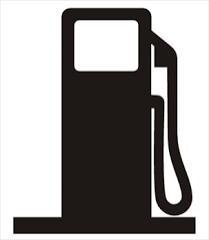Did you find an error or inaccuracy? Feel free to write us. Thank you!Tips to related online calculators
Need help to calculate sum, simplify or multiply fractions? Try our fraction calculator.
Do you have a linear equation or system of equations and looking for its solution? Or do you have a quadratic equation?
Tip: Our volume units converter will help you with the conversion of volume units.

## Related math problems and questions:

• Gasoline tank 2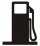A gasoline tank is 1/6 full. When 25 liters of gasoline were added, it became 3/4 full. How many liters are more needed to fill them? Show your solution.
• Petrol as fuel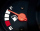A car can travel 480 miles on a full tank of petrol. The tank holds 60 litres. The fuel gauge shows there are 15 litres left in the tank. How many more miles can the car travel before it runs out of petrol?
• A bucketA bucket has 4 liters of water in it when it is 2/5 full. How much can it hold?
• Tank of fuelA 14.5-gallon tank of fuel is 3/4 full. How many more gallons will it take to fill up the tank?
• Tank 11When 150 litres has been drawn from a tank it is 3/8 full, how many litres will the tank hold?
• Fuel tankCarlos has 4/5 of a tank of fuel in his car. He uses 1/10 of a tank per day. How many days will his fuel last?
• Three friendsJohn, Peter, and Pablo each carried a 24 liters bucket full of water down the hill. After they reached the bottom, John's bucket was only 3/4 full, Peter's bucket was 2/3 full, and Pablo's was 1/6 full. How much liters of water did they spill altogether o
• Fractions and mixed numerals(a) Convert the following mixed numbers to improper fractions. i. 3 5/8 ii. 7 7/6 (b) Convert the following improper fraction to a mixed number. i. 13/4 ii. 78/5 (c) Simplify these fractions to their lowest terms. i. 36/42 ii. 27/45 2. evaluate the follow
• Fuel mileage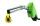You have 3/8 tank of fuel. Estimate fuel consumption @ 6mpg. Estimate speed @ 50mph. Your fuel tank holds 200 gals. Figure out fuel mileage.
• Aircraft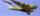The aircraft has in a fuel tank 68 hl of aviation fuel and flight consumes 3.6 liters of fuel. Identify the function, which expresses the dependence of the volume of fuel in tank on the track distance plane flew by. How many hectolites of fuel is still in
• Tom has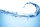Tom has a water tank that holds 5 gallons of water. Tom uses water from a full tank to fill 6 bottles that each hold 16 ounces and a pitcher that holds 1/2 gallon. How many ounces of water are left in the water tank?
• Lenka and money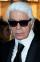When Lenka bought pants from 1/3 of her savings shirt from 1/5 and 1/8 spent at the hairdresser, she was left 328 Kč. How much money was saved? How many hairdressers cost?
• A cylindrical tankA cylindrical tank can hold 44 cubic meters of water. If the radius of the tank is 3.5 meters, how high is the tank?
• Equivalent equationWhich equation, when solved, results in a different value of x than the other three? A. - (7)/(8) x - (3)/(4) = 20 Negative 7/8 x minus three-fourths = 20 B. (3)/(4) + (7)/(8) x= -20 Three-fourths + StartFraction 7 over 8 En
• Fraction eq2/3x + 5/7 = 1/2x + 22/21
• Car range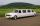Kia has an average diesel consumption of 3.9 liters per 100 kilometers. What is the range of the car for 179 euros if fuel costs 1.3 EUR/liter, if the tank has a volume of 55 liters?
• Fuel consumption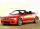Škoda car has an average diesel consumption of 5.9 liters per 100 kilometers. What is the car range for a full tank of volume 70 liters?